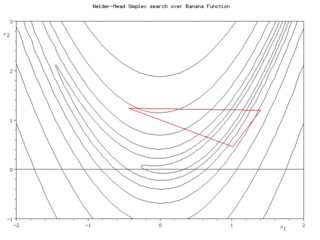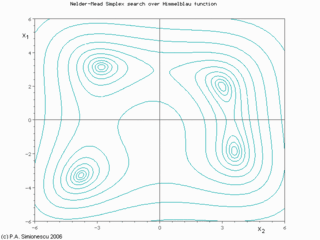Nelder–Mead simplex search over the Rosenbrock banana function (above) and Himmelblau's function (below)
See simplex algorithm for Dantzig's algorithm for the problem of linear optimization.

The Nelder–Mead method or downhill simplex method or amoeba method is a commonly used nonlinear optimization technique, which is a well-defined numerical method for twice differentiable and unimodal problems. However, the Nelder–Mead technique is a heuristic search method that can converge to non-stationary points on problems that can be solved by alternative methods.

The Nelder–Mead technique was proposed by John Nelder & Roger Mead (1965)  and is a technique for minimizing an objective function in a many-dimensional space.

## Overview

The method uses the concept of a simplex, which is a special polytope of N + 1 vertices in N dimensions. Examples of simplices include a line segment on a line, a triangle on a plane, a tetrahedron in three-dimensional space and so forth.

The method approximates a local optimum of a problem with N variables when the objective function varies smoothly and is unimodal.

For example, a suspension bridge engineer has to choose how thick each strut, cable, and pier must be. Clearly these all link together, but it is not easy to visualize the impact of changing any specific element. The engineer can use the Nelder–Mead method to generate trial designs which are then tested on a large computer model. As each run of the simulation is expensive, it is important to make good decisions about where to look.

Nelder–Mead generates a new test position by extrapolating the behavior of the objective function measured at each test point arranged as a simplex. The algorithm then chooses to replace one of these test points with the new test point and so the technique progresses. The simplest step is to replace the worst point with a point reflected through the centroid of the remaining N points. If this point is better than the best current point, then we can try stretching exponentially out along this line. On the other hand, if this new point isn't much better than the previous value, then we are stepping across a valley, so we shrink the simplex towards a better point.

Unlike modern optimization methods, the Nelder–Mead heuristic can converge to a non-stationary point unless the problem satisfies stronger conditions than are necessary for modern methods. Modern improvements over the Nelder–Mead heuristic have been known since 1979.

Many variations exist depending on the actual nature of the problem being solved. A common variant uses a constant-size, small simplex that roughly follows the gradient direction (which gives steepest descent). Visualize a small triangle on an elevation map flip-flopping its way down a valley to a local bottom. This method is also known as the Flexible Polyhedron Method. This, however, tends to perform poorly against the method described in this article because it makes small, unnecessary steps in areas of little interest.

## One possible variation of the NM algorithm

• 1. Order according to the values at the vertices:$f(\textbf{x}_{1}) \leq f(\textbf{x}_{2}) \leq \cdots \leq f(\textbf{x}_{n+1})$
• 2. Calculate xo, the center of gravity of all points except xn + 1.
• 3. Reflection
Compute reflected point$\textbf{x}_r = \textbf{x}_o + \alpha (\textbf{x}_o - \textbf{x}_{n+1})$
If the reflected point is better than the second worst, but not better than the best, i.e.:$f(\textbf{x}_{1}) \leq f(\textbf{x}_{r}) < f(\textbf{x}_{n})$,
then obtain a new simplex by replacing the worst point xn + 1 with the reflected point xr, and go to step 1.
• 4. Expansion
If the reflected point is the best point so far,$f(\textbf{x}_{r}) < f(\textbf{x}_{1}),$
then compute the expanded point$\textbf{x}_{e} = \textbf{x}_o + \gamma (\textbf{x}_o - \textbf{x}_{n+1})$
If the expanded point is better than the reflected point,$f(\textbf{x}_{e}) < f(\textbf{x}_{r})$
then obtain a new simplex by replacing the worst point xn + 1 with the expanded point xe, and go to step 1.
Else obtain a new simplex by replacing the worst point xn + 1 with the reflected point xr, and go to step 1.
Else (i.e. reflected point is not better than second worst) continue at step 5.
• 5. Contraction
Here, it is certain that$f(\textbf{x}_{r}) \geq f(\textbf{x}_{n})$
Compute contracted point$\textbf{x}_{c} = \textbf{x}_{n+1}+\rho(\textbf{x}_{o}-\textbf{x}_{n+1})$
If the contracted point is better than the worst point, i.e.$f(\textbf{x}_{c}) < f(\textbf{x}_{n+1})$
then obtain a new simplex by replacing the worst point xn + 1 with the contracted point xc, and go to step 1.
Else go to step 6.
• 6. Reduction
For all but the best point, replace the point with$x_{i} = x_{1} + \sigma(x_{i} - x_{1}) \text{ for all i } \in\{2,\dots,n+1\}$. go to step 1.

Note: α, γ, ρ and σ are respectively the reflection, the expansion, the contraction and the shrink coefficient. Standard values are α = 1, γ = 2, ρ = 1 / 2 and σ = 1 / 2.

For the reflection, since xn + 1 is the vertex with the higher associated value among the vertices, we can expect to find a lower value at the reflection of xn + 1 in the opposite face formed by all vertices point xi except xn + 1.

For the expansion, if the reflection point xr is the new minimum along the vertices we can expect to find interesting values along the direction from xo to xr.

Concerning the contraction: If f(xr) > f(xn) we can expect that a better value will be inside the simplex formed by all the vertices xi.

The initial simplex is important, indeed, a too small initial simplex can lead to a local search, consequently the NM can get more easily stuck. So this simplex should depend on the nature of the problem.

Wikimedia Foundation. 2010.

### Look at other dictionaries:

• Nelder-Mead method — See simplex algorithm for the numerical solution of the linear programming problem. The Nelder Mead method or downhill simplex method or amoeba method is a commonly used nonlinear optimization algorithm. It is due to John Nelder R. Mead (1965)… …   Wikipedia

• Método Nelder-Mead — Búsqueda del valor mínimo a través del simplex Nelder–Mead en las función banana de Rosenbrock (arriba) y en la función de Himmelblau (abajo) El método Nelder Mead es un algoritmo de optimización ampliamente utilizado. Es …   Wikipedia Español

• Methode de Nelder-Mead — Méthode de Nelder Mead Illustration de la méthode de Nelder Mead La méthode de Nelder Mead est un algorithme d optimisation non linéaire. Elle est publiée par Nelder et Mead en 1965. C est une méthode numérique qui minimise une fonction dans… …   Wikipédia en Français

• Méthode De Nelder-Mead — Illustration de la méthode de Nelder Mead La méthode de Nelder Mead est un algorithme d optimisation non linéaire. Elle est publiée par Nelder et Mead en 1965. C est une méthode numérique qui minimise une fonction dans un espace à plusieurs… …   Wikipédia en Français

• Méthode de nelder-mead — Illustration de la méthode de Nelder Mead La méthode de Nelder Mead est un algorithme d optimisation non linéaire. Elle est publiée par Nelder et Mead en 1965. C est une méthode numérique qui minimise une fonction dans un espace à plusieurs… …   Wikipédia en Français

• Méthode de Nelder-Mead — Illustration de la méthode de Nelder Mead sur la fonction de Rosenbrock La méthode de Nelder Mead est un algorithme d optimisation non linéaire. Elle est publiée par Nelder  …   Wikipédia en Français

• John Nelder — John Ashworth Nelder FRS (born 8 October 1924) is a British statistician.Born in Dulverton, Somerset, he was educated at Blundell s School and Sidney Sussex College, Cambridge, where he read mathematics.Nelder s appointments include Head,… …   Wikipedia

• Downhill-Simplex-Verfahren — Der Simplex Algorithmus nach John Nelder und Roger Mead (Comp. J., vol. 7, 1965, p. 308) oder auch Downhill Simplex Verfahren oder manchmal auch einfach Simplex Algorithmus ist im Unterschied zum Namensvetter für lineare Probleme (Simplex… …   Deutsch Wikipedia

• Simplex algorithm — In mathematical optimization theory, the simplex algorithm, created by the American mathematician George Dantzig in 1947, is a popular algorithm for numerical solution of the linear programming problem. The journal Computing in Science and… …   Wikipedia

• List of numerical analysis topics — This is a list of numerical analysis topics, by Wikipedia page. Contents 1 General 2 Error 3 Elementary and special functions 4 Numerical linear algebra …   Wikipedia